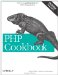# Recipe 6.5. Creating Functions That Take a Variable Number of Arguments

### Recipe 6.5. Creating Functions That Take a Variable Number of Arguments

#### 6.5.1. Problem

You want to define a function that takes a variable number of arguments.

#### 6.5.2. Solution

Pass an array and place the variable arguments inside the array:

`// find the "average" of a group of numbers function mean(\$numbers) {     // initialize to avoid warnings     \$sum = 0;     // the number of elements in the array     \$size = count(\$numbers);     // iterate through the array and add up the numbers     for (\$i = 0; \$i < \$size; \$i++) {         \$sum += \$numbers[\$i];     }     // divide by the amount of numbers     \$average = \$sum / \$size;     // return average     return \$average; } \$mean = mean(array(96, 93, 97));`

#### 6.5.3. Discussion

There are two good solutions, depending on your coding style and preferences. The more traditional PHP method is the one described in the Solution. We prefer this method because using arrays in PHP is a frequent activity; therefore, all programmers are familiar with arrays and their behavior.

So while this method creates some additional overhead, bundling variables is commonplace. It's done in Recipe 6.4 to create named parameters and in Recipe 6.7 to return more than one value from a function. Also, inside the function, the syntax to access and manipulate the array involves basic commands such as \$array[\$i] and count(\$array).

However, this can seem clunky, so PHP provides an alternative and allows you direct access to the argument list, as shown in Example 6-1.

##### Accessing function parameters without using the argument list

 `// find the "average" of a group of numbers function mean() { // initialize to avoid warnings \$sum = 0; // the number of arguments passed to the function \$size = func_num_args(); // iterate through the arguments and add up the numbers for (\$i = 0; \$i < \$size; \$i++) { \$sum += func_get_arg(\$i); } // divide by the amount of numbers \$average = \$sum / \$size; // return average return \$average; } \$mean = mean(96, 93, 97);`

This example uses a set of functions that return data based on the arguments passed to the function they are called from. First, func_num_args( ) returns an integer with the number of arguments passed into its invoking function'in this case, mean( ). From there, you can then call func_get_arg( ) to find the specific argument value for each position.

When you call mean(96, 93, 97), func_num_args( ) returns 3. The first argument is in position 0, so you iterate from 0 to 2, not 1 to 3. That's what happens inside the for loop where \$i goes from 0 to less than \$size. As you can see, this is the same logic used in Example 6-1 in which an array was passed. If you're worried about the potential overhead from using func_get_arg( ) inside a loop, don't be. This version is actually faster than the array passing method.

There is a third version of this function that uses func_get_args( ) to return an array containing all the values passed to the function. It ends up looking like a hybrid between the previous two functions, as shown in Example 6-2.

##### Accessing function parameters without using the argument list

 `// find the "average" of a group of numbers function mean() { // initialize to avoid warnings \$sum = 0; // load the arguments into \$numbers \$numbers = func_get_args(); // the number of elements in the array \$size = count(\$numbers); // iterate through the array and add up the numbers for (\$i = 0; \$i < \$size; \$i++) { \$sum += \$numbers[\$i]; } // divide by the amount of numbers \$average = \$sum / \$size; // return average return \$average; } \$mean = mean(96, 93, 97);`

Here you have the dual advantages of not needing to place the numbers inside a temporary array when passing them into mean( ), but inside the function you can continue to treat them as if you did. Unfortunately, this method is slightly slower than the first two.

Recipe 6.7 on returning multiple values from a function; documentation on func_num_args( ) at http://www.php.net/func-num-args, func_get_arg( ) at http://www.php.net/func-get-arg, and func_get_args( ) at http://www.php.net/func-get-args.PHP Cookbook: Solutions and Examples for PHP Programmers
ISBN: 0596101015
EAN: 2147483647
Year: 2006
Pages: 445